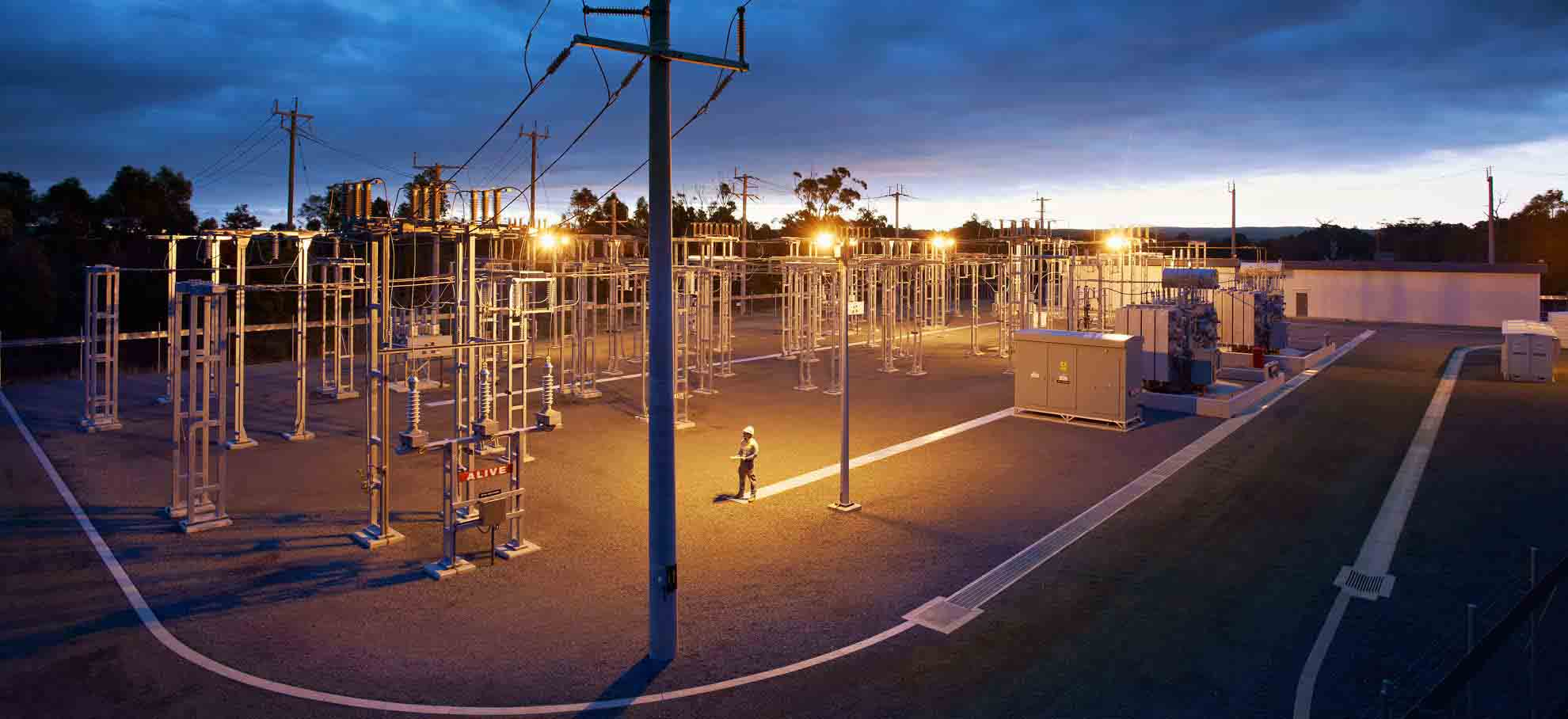# MATLAB/Simulink Course: Power System Simulations (Load Flow, Short Circuit & Stability)

Learn how to simulate power systems in MATLAB/Simulink by building several Simulink power system models for load flow, short circuit, and power stability studies) and analyze the behavior of the system under several conditions.

## Course Overview

This course is designed to allow you to simulate power systems in MATLAB/Simulink. This course not only gives a review of the theory of how power systems operate but also gives several examples of how to run different types of power system studies using MATLAB/Simulink.

The course is divided into the following sections:

### 1. Introduction to MATLAB/Simulink for Power Systems

In the first section of the course, we will begin by reviewing the libraries available in Simulink to represent generators, transformers, transmission lines, and loads in our models. After that, we will take a look at how we can model these components in Simulink, as well as how we can put them together in a model and how we can take measurements in the model to ensure proper simulation.

### 2. Power System Studies in MATLAB/Simulink

After we’ve made ourselves familiar with the MATLAB/Simulink environment building a small power system model, we will move on to build a large power system model which includes several generators, transformers, transmission lines, loads, and capacitor banks. We will also model the turbine control systems and excitation control systems for all generators to simulate the realistic dynamic behavior of power systems in real life.

After we have built the entire model, we will run several types of studies, including load flow, short circuit, and stability studies, to simulate the behavior of the system under several conditions. This will give us all the tools we need to build any type of power system and run any power system study using MATLAB/Simulink.

As mentioned above, in each section, we will go over several models to illustrate how we can design and simulate power systems in MATLAB/Simulink. The models are also available for download so that you can follow along, as well as use these models and modify them to create your own power system models.

By learning how to simulate power systems in MATLAB/Simulink, you will be able to further your career in electrical engineering and power engineering.

## Course Overview

• How to simulate power systems in MATLAB/Simulink
• How to build power system models
• How to simulate faults
• How to take measurements in MATLAB/Simulink to ensure proper simulation
• How to model generators, transformers, transmission lines, capacitor banks, and loads in MATLAB/Simulink
• How to run power flow (load flow) studies
• How to simulate the dynamic behavior of power systems
• Power Engineering and Electrical Engineering simulations in MATLAB/Simulink

## Who Is This Course For

• Engineering students
• Practicing Engineers
• Anybody with an interest in learning about power systems and/or MATLAB/Simulink

## Requirements

• MATLAB/Simulink software, free trial available online

• Kundur_Two_Area_Power_System_S03 (zip)
• Example_Power_System_Model_S02 (zip)
• Kundur Two-Area Power System_S03 (xlsx)
• Example Power System Data_S02 (xlsx)
• Transmission Line Data_S02 (pdf)
• Transformer Data_S02 (pdf)
• Thevenin Equivalent Data_S02 (pdf)
• One-line Diagram_S03 (pdf)
• MATLAB-Simulink for Power System Simulations Course Outline (pdf)
• Generator Data_S02 (pdf)
• Data for the Turbine and Excitation Control Systems_S03 (pdf)
• Data for the Transmission Lines_S03 (pdf)
• Data for the Transformers_S03 (pdf)
• Data for the System Equivalent_S03 (pdf)
• Data for the Loads_S03 (pdf)
• Data for the Generators_S03 (pdf)
• Data for the Capacitor Banks_S03 (pdf)

## Course Content

Lesson Content
0% Complete 0/3 Steps
Lesson Content
0% Complete 0/8 Steps

##### Ricardo Romero, PE

Hi, my name is Ricardo Romero and I am an Electrical Engineer with over ten years of experience in Power Systems. I obtained my Bachelor's and Master's degrees in Electrical Engineering from The University of North Carolina at Charlotte. I am also a licensed Professional Engineer in Power Systems in the states of Arizona, Louisiana, North Carolina, Pennsylvania, South Carolina, and Virginia in the United States. Although my professional experience is mainly in Power Systems, I am also passionate about other topics in Electrical Engineering including Analog Electronics, Control Systems, Digital Design, Digital Signal Processing, Electric Machinery, and Power Electronics.

13 Courses+168 enrolled
Not Enrolled

#### Course Includes

• 3 Lessons
• 21 Topics
• 1 Quiz
• Course Certificate